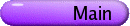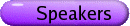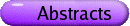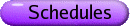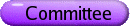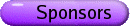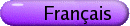L-functions, Automorphic Forms and Representation Theory
Org: Amir Akbary (Lethbridge) and Clifton Cunningham (Calgary)
[PDF]

JEFFREY ACHTER, Colorado State University, Fort Collins, CO 80523, USA
Monodromy representations and function field class numbers
[PDF]

Given an elliptic curve over a finite field, one might ask for the chance that it has a rational point of order l. More generally, what is the chance that a curve drawn from a family over a finite field has a point of order l on its Jacobian?

The answer is encoded in the l-adic monodromy representation of the family in question. In this talk, I'll discuss recent work on this representation for various families of curves, and use it to prove a Cohen-Lenstra-type result for class groups of function fields.

IMIN CHEN, Simon Fraser University
Diophantine equations via Galois representations
[PDF]

Recently, the use of Galois representations attached to elliptic curves has been used to resolve several cases of the generalized Fermat equation. In this talk, I will discuss the method and some further cases which can be analyzed at least partially, including the equation a2+ b2p = cr, where r = 3 or 5. Although a complete resolution is not yet possible, a computational criterion can be obtained for r = 3, based on previous work by Bennett-Skinner and Kraus. For r = 5, I outline a possible strategy using a combination of quadratic Q-curves and elliptic curves over Q.

GERALD CLIFF, University of Alberta, Dept. of Math. and Stat. Sci., Edmonton, AB
K-types of local Weil representations
[PDF]

Let F be a nonarchimedian local field with ring of integers R, maximal ideal P, and residue field k of odd characteristic. Let W be the Weil representation of the symplectic group Sp(2n,F), corresponding to a character c of the additive group of F. Suppose that the conductor of c is the fractional ideal Pl. If l is even, the restriction of W to the maximal compact subgroup Sp(2n,R) is known to be a direct sum Åm=0¥ Tm, where each Tm is can be regarded as a representation of Sp(2n,R/P2m); this uses the lattice model of the Schrödinger representation of the Heisenberg group. We show that there is an analogous decomposition in the case that l is odd. Each Tm arises as a direct summand of a Weil-like representation of Sp(2n,R/P2m+1). In particular, T0 is the Weil representation of Sp(2n,k).

This is joint work with David McNeilly.

CHANTAL DAVID, Concordia University
Non-vanishing of cubic twists of elliptic curves
[PDF]

We will present a proof of a quantitative non-vanishing result for cubic twists of an elliptic curve E over the field K = Q(Ö{-3}). Our proof is based on the classical approach of Iwaniec (for the case of quadratic twists of L-functions). A similar result was also obtained by Brubaker, Friedberg and Hoffstein by studying some multiple Dirichlet series. We obtain that at least X2/3-e twisted L-functions do not vanish at the critical point. If one could improve the large sieve inequality of Heath-Brown for cubic characters by removing a residual term, the proportion of non-vanishing would be at least X1-e, which is the optimal result that can be obtained without mollifying.

Joint work with D. Milicevic and G. Ricotta.

LASSINA DEMBELE, University of Calgary
Examples of automorphic forms on the unitary group U(3)
[PDF]

In this talk, we will present examples of automorphic forms on the unitary groups in three variables attached to various quadratic extensions E/F. We will then study their corresponding Galois representations. Namely, we will determine the set of exceptional primes which corresponds to the p-adic family attached to each of the forms. Those sets which are very important for arithmetic applications, have been determined for classical modular forms by works of Serre, Ribet and others. But there is no known result in the case of the U(3).

WENTANG KUO, University of Waterloo, Waterloo, Ontario
A generalization of the Sato-Tate Conjecture
[PDF]

The original Sato-Tate Conjecture concerns the angle distribution of the eigenvalues arisen from non-CM elliptic curves. In this talk, we formulate an analogue of the Sato-Tate Conjecture for generic automorphic forms of GLn and conjecture their angle distributions. Under a reasonable hypothesis, we can prove that the expected distribution is indeed true.

YU-RU LIU, University of Waterloo, Waterloo, Ontario
On Erdös-Pomerance's conjecture for the Carlitz module
[PDF]

For a Î Z, m Î N with (a,m)=1, let la(m) be the order of a in (Z/mZ)*. Let w( la(m) ) be the number of distinct prime divisors of la(m). A conjecture of Erdös and Pomerance states that if |a| > 1, then the quantity

 w æè la(m) öø - 1 2 (loglogm)2

 1 Ö3 (loglogm)3/2
distributes normally. The problem remains open until today. A conditional proof of it was obtained recently by Murty and Saidak. Li and Pomerance also provided an alternative proof of the same result. In this talk, we formulate an analogous question for the Carlitz module and provide an unconditional proof of it.

This is a joint work with W. Kuo.

PAUL MEZO, Carleton University, 1125 Colonel By Drive, Ottawa, ON K1S 5B6
Twisted trace Paley-Wiener theorems
[PDF]

One method of comparing automorphic L-functions arising from different groups is the comparison of trace formulas. Occasionally there is a group automorphism which presents itself in the comparison of "twisted" trace formulas. To implement the automorphism properly, one must characterize twisted characters at the archimedean places. We describe recent progress in this characterization, which is joint work with P. Delorme.

FIONA MURNAGHAN, University of Toronto
Tame supercuspidal representations
[PDF]

We will discuss recent results (joint with Jeff Hakim) concerning criteria for equivalence of tame supercuspidal representations. These criteria are expressed in terms of the G-data used in J.-K. Yu's construction of tame supercuspidal representations. Then we will indicate how the criteria for equivalence can be used to give a new parametrization of tame supercuspidal representations in some cases.

KUMAR MURTY, Toronto

RAM MURTY, Queen's University
The Lang-Trotter conjecture
[PDF]

Given a normalized Hecke eigenform f of weight k and level N, let an(f) denote its n-th Fourier coefficient. The generalized Lang-Trotter conjecture predicts that for k > 3, and a given value c, the number of n such that an(f) = c, is finite. If c is odd and N=1, we will prove this conjecture. By connecting this to Serre's epsilon conjecture, we resolve it for all levels of the form 2a N0 with N0 = 1, 3, 5, 15 or 17.

This is joint work with V. Kumar Murty.

NATHAN NG, University of Ottawa
Discrete mean values of the Riemann zeta function
[PDF]

I will survey the theory of the discrete moments of the Riemann zeta function and I will indicate how they differ from the ordinary continuous moments of the zeta function. In addition, I will highlight several number theoretic applications that can be derived from knowing asymptotics for the discrete moments. In particular, we will show that there exist large and small values of z¢(r) where r denotes a non-trivial zero of the Riemann zeta function.

RACHEL PRIES, Colorado State University, Fort Collins, CO
Twists of representations of fundamental groups in positive characteristic
[PDF]

We study a twisting action on representations of fundamental groups of affine varieties in positive characteristic. For affine curves, we study how this twisting affects invariants of the representation. This yields applications to the subject of Galois covers of curves in positive characteristic.

HADI SALMASIAN, Queen's University, Kingston, ON
Small degenerate principal series and exceptional dual pairs
[PDF]

I will describe a general theorem which connects unitary representations of reductive groups and Kirillov's orbital theory for nilpotent groups. We show how this result applies to the study of certain dual pairs in exceptional groups which basically generalize the (noncompact) GL(1)-GL(n) duality.